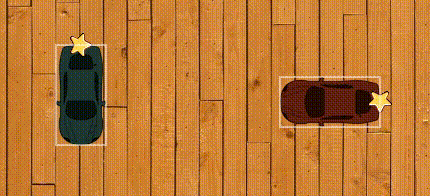# P2 Physic collide while not overlapped

## Recommended Posts

Hi there,

I'm working on some P2 physic and getting the attached result, which is weird because the two objects collides without overlapping on one another.

I'm I missing something?

The code is as follow:

``````      game.physics.enable(car, Phaser.Physics.P2JS);
car.body.debug = true
car.body.angle = car.angle
car.body.rotation = car.rotation

car.body.setCollisionGroup(this.carCollisionGroup);
var v =100;
var angle = car.body.angle;

var vx = v * Math.cos(angle * (Math.PI/180))
var vy = v * Math.sin(angle * (Math.PI/180))

car.body.velocity.x = vx;
car.body.velocity.y = vy;``````

Thanks##### Share on other sites

Hi,

Sorry for the delay, I only work on this on weekend so haven't had a chance to follow up.

Here is an extraction of my app:

You may need to refresh the page a few times for the cars to show up. Just hit the play button.

Here is the code, thank you so much:

``````<!doctype html>
<html>
<meta charset="UTF-8" />
<title>hello phaser!</title>
<script type="text/javascript" src="https://cdn.jsdelivr.net/phaser/2.5.0/phaser.js"></script>
<body>

<div id='main'>
</div>

<script type="text/javascript">
var TheGame = {
Params : {
iconSize: 364,
}
}

var BootState = {
init : function(){
this.scale.scaleMode = window.Phaser.ScaleManager.RESIZE;
this.game.stage.backgroundColor = "#f8f8f8";
},

},

create : function(){
this.state.start("ScenarioSetup");
this.input.touch.preventDefault = false;
this.game.physics.startSystem(window.Phaser.Physics.P2JS);
this.game.physics.p2.setImpactEvents(true);
},
}

var ScenarioSetup = {
this.cars = []
},

create : function() {
var game = this.game

this.background.height = game.height;
this.background.width = game.width;

this.carsGroup.enableBody = true;
this.carsGroup.physicsBodyType = window.Phaser.Physics.P2JS;

this.carsCollisionGroup = game.physics.p2.createCollisionGroup();

var scenario = [
{"x":301.2662461222614,"y":243.0936843225281,"rotation":-1.616663179600873,"setSpeed":"0","setAngle":0,"tint":12854726},
{"x":592.8282701605795,"y":240.70069280486896,"rotation":3.1072777136041276,"setSpeed":"80","setAngle":0,"tint":12854726}
]
if (scenario && scenario.length !== 0){
for (var i = 0; i < scenario.length; i++){
var savedCar = scenario[i]
this.createCar(savedCar.x, savedCar.y, savedCar.tint, savedCar.rotation, savedCar.setSpeed, savedCar.setAngle)
}
}
},

play : function(){
if (this.isPlaying){
return
}
if (this.cars.length === 0){
return
}

var game = this.game
var Phaser = window.Phaser

for (var i = 0; i < this.cars.length; i++){
var car = this.cars[i]

game.physics.enable(car, Phaser.Physics.P2JS);

car.body.angle = car.angle
car.body.rotation = car.rotation

car.body.setCollisionGroup(this.carsCollisionGroup);
car.body.collides(this.carsCollisionGroup, function(){}, car);

var v = car.setSpeed*4;
var angle = car.body.angle;

var vx = v * Math.cos(angle * (Math.PI/180))
var vy = v * Math.sin(angle * (Math.PI/180))

car.body.velocity.x = vx;
car.body.velocity.y = vy;
}
this.isPlaying = true
},

createCar : function(x,y,tint,rotation, speed, angle){
var game = this.game
var car = game.add.sprite(x, y, "car");
car.tint = tint;
car.inputEnabled = true;
car.input.enableDrag(true);
car.anchor.setTo(0.5,0.5)
car.setSpeed = speed
car.setAngle = angle
car.rotation = rotation
this.cars.push(car)

return car
},
}

var game = new Phaser.Game(800, 600, Phaser.CANVAS, 'main');
game.state.start("Boot");
};
</script>
</body>
</html>``````

##### Share on other sites

The problem is that you are mixing up the angles and rotations of the p2 body. Its better to stick to either using angles(degrees) or rotations(radians).

in the for loop you can use this:

``````            for (var i = 0; i < this.cars.length; i++){
var car = this.cars[i]

game.physics.p2.enable(car,true);
//car.body.rotation=car.originalRotation;//either this
car.body.angle=car.originalAngle;//or this
car.body.setCollisionGroup(this.carsCollisionGroup);
car.body.collides(this.carsCollisionGroup, function(){}, car);

var v = car.setSpeed*4;
var angle = car.body.angle;

var vx = v * Math.cos(angle * (Math.PI/180))
var vy = v * Math.sin(angle * (Math.PI/180))

car.body.velocity.x = vx;
car.body.velocity.y = vy;
}``````

and in createCar set a variable that is not named rotation or angle:

``````          createCar : function(x,y,tint,rotation, speed, angle){
var game = this.game
var car = game.add.sprite(x, y, "car");
car.tint = tint;
car.inputEnabled = true;
car.input.enableDrag(true);
car.anchor.setTo(0.5,0.5)
car.setSpeed = speed;
car.originalAngle=angle;//car.angle would set the angle of the sprite
car.originalRotation=rotation; //car.rotation would set the rotation of the sprite
this.cars.push(car)
return car
},``````

Here is a working version:

``````<!doctype html>
<html>
<meta charset="UTF-8" />
<title>hello phaser!</title>
<script type="text/javascript" src="https://cdn.jsdelivr.net/phaser/2.5.0/phaser.js"></script>
<body>

<div id='main'>
</div>

<script type="text/javascript">
var TheGame = {
Params : {
iconSize: 364,
}
}

var BootState = {
init : function(){
this.scale.scaleMode = window.Phaser.ScaleManager.RESIZE;
this.game.stage.backgroundColor = "#f8f8f8";
},

},

create : function(){
this.state.start("ScenarioSetup");
this.input.touch.preventDefault = false;
this.game.physics.startSystem(window.Phaser.Physics.P2JS);
this.game.physics.p2.setImpactEvents(true);
},
}

var ScenarioSetup = {
this.cars = []
},

create : function() {
var game = this.game

this.background.height = game.height;
this.background.width = game.width;

this.carsGroup.enableBody = true;
this.carsGroup.physicsBodyType = window.Phaser.Physics.P2JS;

this.carsCollisionGroup = game.physics.p2.createCollisionGroup();

var scenario = [
{"x":301.2662461222614,"y":243.0936843225281,"rotation":1.57,"setSpeed":"0","setAngle":90,"tint":12854726},
{"x":592.8282701605795,"y":240.70069280486896,"rotation":0,"setSpeed":"-80","setAngle":0,"tint":12854726}
]
if (scenario && scenario.length !== 0){
for (var i = 0; i < scenario.length; i++){
var savedCar = scenario[i]
this.createCar(savedCar.x, savedCar.y, savedCar.tint, savedCar.rotation, savedCar.setSpeed, savedCar.setAngle)
}
}
},

play : function(){
if (this.isPlaying){
return
}
if (this.cars.length === 0){
return
}

var game = this.game
var Phaser = window.Phaser

for (var i = 0; i < this.cars.length; i++){
var car = this.cars[i]

game.physics.p2.enable(car,true);
//car.body.rotation=car.originalRotation;//either this
car.body.angle=car.originalAngle;//or this
car.body.setCollisionGroup(this.carsCollisionGroup);
car.body.collides(this.carsCollisionGroup, function(){}, car);

var v = car.setSpeed*4;
var angle = car.body.angle;

var vx = v * Math.cos(angle * (Math.PI/180))
var vy = v * Math.sin(angle * (Math.PI/180))

car.body.velocity.x = vx;
car.body.velocity.y = vy;
}
this.isPlaying = true
},

createCar : function(x,y,tint,rotation, speed, angle){
var game = this.game
var car = game.add.sprite(x, y, "car");
car.tint = tint;
car.inputEnabled = true;
car.input.enableDrag(true);
car.anchor.setTo(0.5,0.5)
car.setSpeed = speed;
car.originalAngle=angle;
car.originalRotation=rotation;
this.cars.push(car)

return car
},
}

var game = new Phaser.Game(800, 600, Phaser.CANVAS, 'main');
game.state.start("Boot");
};
</script>
</body>
</html>``````

##### Share on other sites

Thank you very much. With a little tweak, I was also able to make it look correct right from the beginning.

Much appreciated